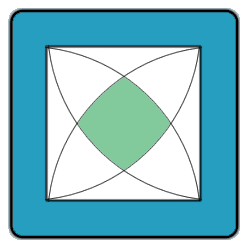All about flooble | fun stuff | Get a free chatterbox | Free JavaScript | Avatarsperplexus dot infoQuarter intersection Problem (Posted on 2002-10-30)Below is an image of a square, 7 cm to a side, with four quarter-circles inscribed into it as showm.

Find the area of the shaded region in the center.See The Solution Submitted by Dulanjana Rating: 4.0667 (15 votes)Comments: ( Back to comment list | You must be logged in to post comments.)Solution| Comment 8 of 9 |Imagine only the bottom side of the square and two arcs with their centers on the endpoints of the refered bottom side. The arcs intersect forming a characteristic of an imaginary equilatteral triangle. From the left vertex of the square to the intersection of the arcs, it has an inclination of 60 degrees. The diagonal from opposite vertex in the square, has an inclination of 45 degrees, so 60-45 = 15 degrees, we form a right triangle of one angle equal to 15 and an hypotenusa equals to the radius of one arc, that is 7cm. With this triangle we obtain the two sides (7*Sin(15) and 7*Cos(15)), these sides will help us to obtain the proper areas in order to obtain the final of the shaded region. The shaded region is composed by the area of a square with a side equal to 2*7*sin(15) and 4 circular sections that are very small, but they all count. These circular sections are obtained by first obtaining the linear relation of the proportional area that the circular segments relate to the total area of the circle with a radius of 7cm. We subtract an isoceles triangle formed by a side equal to 2*7*sin(15) and two equal sides of 7cm. To the proportional area of the small circle segment we subtract the mentioned isoceles triangle and we get one of the four circle segment areas. So we multiply this area times 4 and add the area previously calculated of the square of side 2*7*sin(15).
Area = (49/3)*(12*sin(15)*(sin(15)-cos(15))+Pi) = 15.4421904378 cm²
Note: I did not write how to obtain the area of the isoceles triangle or the value of one of the four circle segment areas, I posted my final ecuation of the total area, so any area obtained is within the final ecuation.
 Posted by Antonio on 2003-08-31 06:00:14Please log in:

 Search: Search body:
Forums (0)